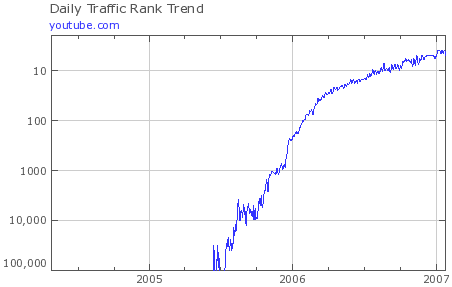Search IntMath
Close

# Interesting semi-logarithmic graph - YouTube Traffic Rank

By Murray Bourne, 25 Jan 2007

The growth in popularity of YouTube has been extraordinary. It has gone from an unknown, unheard-of site (not even in the top 100,000 sites, so off the scale) to the 5th highest ranked site on the Web, all in the space of 18 months.

This image, from Alexa, is an interesting example of a semi-logarithmic graph. The vertical scale (traffic rank) is logarithmic, while the horizontal scale (time) is linear.### Comment Preview

HTML: You can use simple tags like <b>, <a href="...">, etc.

To enter math, you can can either:

1. Use simple calculator-like input in the following format (surround your math in backticks, or qq on tablet or phone):
a^2 = sqrt(b^2 + c^2)
(See more on ASCIIMath syntax); or
2. Use simple LaTeX in the following format. Surround your math with $$ and $$.
$$\int g dx = \sqrt{\frac{a}{b}}$$
(This is standard simple LaTeX.)

NOTE: You can mix both types of math entry in your comment.